# Test Prep Plan - Take a practice test

Take this practice test to check your existing knowledge of the course material. We'll review your answers and create a Test Prep Plan for you based on your results.
How Test Prep Plans work
1
2Based on your results, we'll create a customized Test Prep Plan just for you!
3Study smarter
Study more effectively: skip concepts you already know and focus on what you still need to learn.

# ASVAB Math: Quadratic Equations Chapter Exam

Exam Instructions:

Choose your answers to the questions and click 'Next' to see the next set of questions. You can skip questions if you would like and come back to them later with the yellow "Go To First Skipped Question" button. When you have completed the practice exam, a green submit button will appear. Click it to see your results. Good luck!

### Page 1

#### Question 2 2. Which of the following are the solutions to the equation below?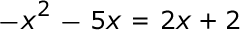#### Question 5 5. Use the quadratic formula to find the solutions to the equation below.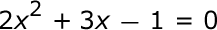### Page 2

#### Question 9 9. Rewrite the quadratic below in standard form: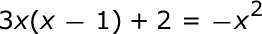### Page 3

#### Question 13 13. Solve this inequality.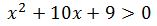#### Question 15 15. Find the roots of the equation.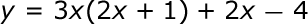### Page 4

#### Question 16 16. What are the zeros in the following quadratic equation?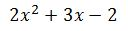#### Question 17 17. Solve the following inequality.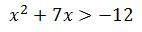#### Question 18 18. Which statement is true about the inequality below?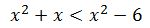#### Question 19 19. Solve the following equation.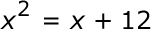#### Question 20 20. Find the roots of the equation below.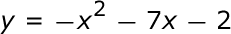### Page 5

#### Question 21 21. What is the b-value of the quadratic equation below?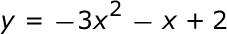#### ASVAB Math: Quadratic Equations Chapter Exam Instructions

Choose your answers to the questions and click 'Next' to see the next set of questions. You can skip questions if you would like and come back to them later with the yellow "Go To First Skipped Question" button. When you have completed the practice exam, a green submit button will appear. Click it to see your results. Good luck!

Support